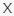September 23, 2023
Latest:
QuizDiva

# Picture Clues Quiz QuizDiva

Q 1. UNSCRAMBLE THE LETTERS TO SEE WHAT THE PICTURES ARE TRYING TO SAY

Choices:

B L A G D U Y
L M A F Y
N G H O I R T M L
Y E T B B E O L E

B L A G D U Y

Q 2. UNSCRAMBLE THE LETTERS TO SEE WHAT THE PICTURES ARE TRYING TO SAY

Choices:

T V N A E U R S
P I E T N L M C A
M F Y O S U L E
S M R A U E O P T

S M R A U E O P T

Q 3. UNSCRAMBLE THE LETTERS TO SEE WHAT THE PICTURES ARE TRYING TO SAY

Choices:

C S I C K T D A N Y
Y C N N E A C D A
C E W A P R P A E N
E S E T C E W A N

Y C N N E A C D A

Q 4. UNSCRAMBLE THE LETTERS TO SEE WHAT THE PICTURES ARE TRYING TO SAY

Choices:

R S E P A A C K E R
R I C A R H N O R A
N O N V A H R
R R O A C H N

R R O A C H N

Q 5. UNSCRAMBLE THE LETTERS TO SEE WHAT THE PICTURES ARE TRYING TO SAY

Choices:

G O L F R D A Y N
Z L R I A D L F Y
W G D O G R G S N A N I
G B D A R U O N G

G O L F R D A Y N

Q 6. UNSCRAMBLE THE LETTERS TO SEE WHAT THE PICTURES ARE TRYING TO SAY

Choices:

I S X A C T W K
H B A X W R A H C
C K C D A E S T N L I
O D C D L O N A E W

C K C D A E S T N L I

Q 7. UNSCRAMBLE THE LETTERS TO SEE WHAT THE PICTURES ARE TRYING TO SAY

Choices:

N U S T S I K C
N U N H O E Y S
S M I C T O N O K
Y O H N O M E O N

Y O H N O M E O N

Q 8. UNSCRAMBLE THE LETTERS TO SEE WHAT THE PICTURES ARE TRYING TO SAY

Choices:

I G N H A R E
O P H O H R A E
R E R N A I G
R R L J A E E W E Y